# Parabolic-equation method

(diff) ← Older revision | Latest revision (diff) | Newer revision → (diff)

A method for the approximate solution of a high-frequency diffraction problem (see Diffraction, mathematical theory of). As a rule it is necessary to resort to the parabolic-equation method for the determination of a wave field in those domains where the ray method cannot be employed because the field of rays suffers from a singularity in one sense or another. Consider, for example, the incidence of a plane wave on a perfectly reflecting convex body. The wave phenomenon is described by the Helmholtz equation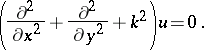(1)

Here the point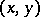is located outside the bounded convex domain, on the boundary of which the Dirichlet boundary condition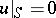is satisfied. It is assumed that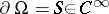has everywhere positive curvature. The solutioncan be represented as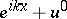, where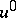satisfies the radiation conditions. The solution of such a problem exists and is unique.

In the high-frequency range ("large" ) it is important to construct a formal small wavelength solution to this problem (i.e. roughly speaking, an expansion that formally satisfies all the conditions of the problem, while successive terms are of increasingly high order of smallness as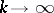). It is possible to show that, in the case under consideration, a formal solution will be an asymptotic expansion of the classical solution.

The ray method allows one to construct the unknown small wavelength expansion everywhere, except in the shadow zone (see Fig.). Rays of the incident wave shadow penumbra region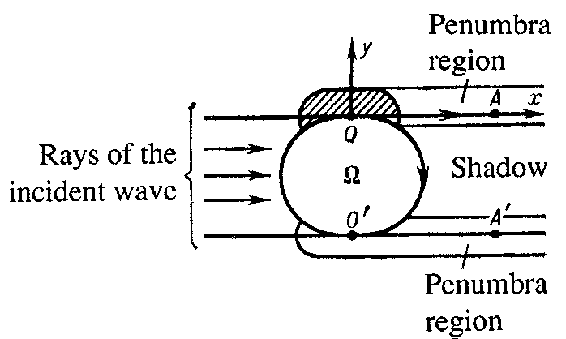Figure: p071200a

The expression for the wave field constructed with the aid of the ray method loses smoothness on the boundary between shadow zone and illuminated zone (the half-linesand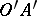in the figure). In a neighbourhood of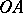(and) the small wavelength asymptotic expansion of the wavefield is no longer given by the ray formulas. A neighbourhood of(and) is usually called a penumbra region.

Of key importance for the construction of a formal solution to the problem given above is an examination of the points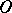and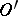where the rays of the incident wave are the boundary curve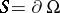. The pointis taken as the origin of coordinates, and the positive-axis separates the shadow zone and the illuminated zone.

One introduces new coordinatesandin a neighbourhood of. A point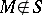is determined by its distance alongfrom. It is assumed that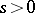(respectively,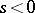) in the shadow zone (respectively, in the illuminated zone). If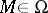, the pointis characterized by its distancefromand the coordinatesof the projection ofon. With respect to the coordinatesand, the Helmholtz equation takes the form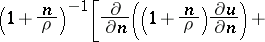(2)(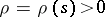is the radius of curvature ofat the point). Through the MacLaurin series for,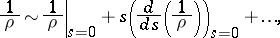it is possible to replace all the coefficients of equation (2) by their formal expansions in powers ofand. Substituting the coordinatesin equations (2), one arrives at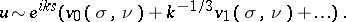(3)

By equating to zero the coefficients of the successive powers of, one obtains the following sequence of recurrence relations (which is typical for the method of boundary layers, cf. also Boundary-layer theory):Here the first equation is a "parabolic" equation, leading to the name parabolic-equation method: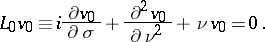(4)

Basically, equation (4) is an equation of Schrödinger type. The coefficients in the operatorare polynomials inand. For the formal satisfaction of the boundary conditionit is sufficient to require that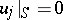. The other boundary conditions require that for large,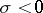, the sequence (3) formally becomes the expansion of the ray method. For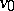it is possible to derive an explicit formula (the so-called Fock formula), having the form of a Fourier integral: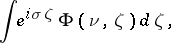whereis expressed comparatively simply in terms of Airy functions. Methods of matching asymptotics enable one to obtain formulas for the wave field in all shadow and penumbra regions.

In the case of creeping waves and of whispering gallery modes, corresponding "parabolic" equations have been deduced and their solutions are expressed in terms of Airy functions. The development of the theory of lasers made it necessary to consider waves concentrated in a neighbourhood of isolated rays. Selecting the corresponding phase coefficient and carrying out further constructions analogous to the constructions of the boundary-layer method, a "parabolic" equation is obtained, in terms of the solution of which the wave field is expressed in a first approximation. In this case the "parabolic" equation will be the Schrödinger equation with a quadratic potential. The parabolic-equation method finds application also in the calculation of the wave field in shallow water, in statistical non-homogeneous media and in many other problems. Analogues of the parabolic-equation method are used in the theory of non-linear waves.

How to Cite This Entry:
Parabolic-equation method. Encyclopedia of Mathematics. URL: http://encyclopediaofmath.org/index.php?title=Parabolic-equation_method&oldid=15772
This article was adapted from an original article by V.M. Babich (originator), which appeared in Encyclopedia of Mathematics - ISBN 1402006098. See original article